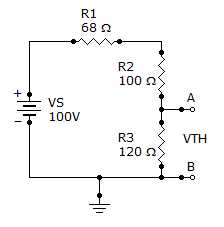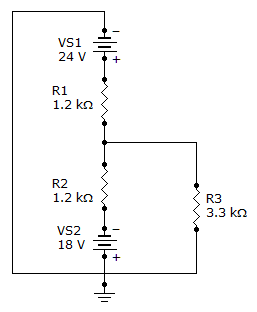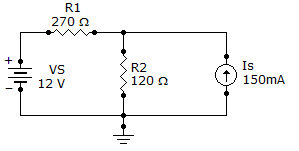Electrical Engineering - Circuit Theorems and Conversions

Why Electrical Engineering Circuit Theorems and Conversions?

In this section you can learn and practice Electrical Engineering Questions based on "Circuit Theorems and Conversions" and improve your skills in order to face the interview, competitive examination and various entrance test (CAT, GATE, GRE, MAT, Bank Exam, Railway Exam etc.) with full confidence.

Where can I get Electrical Engineering Circuit Theorems and Conversions questions and answers with explanation?

IndiaBIX provides you lots of fully solved Electrical Engineering (Circuit Theorems and Conversions) questions and answers with Explanation. Solved examples with detailed answer description, explanation are given and it would be easy to understand. All students, freshers can download Electrical Engineering Circuit Theorems and Conversions quiz questions with answers as PDF files and eBooks.

Where can I get Electrical Engineering Circuit Theorems and Conversions Interview Questions and Answers (objective type, multiple choice)?

Here you can find objective type Electrical Engineering Circuit Theorems and Conversions questions and answers for interview and entrance examination. Multiple choice and true or false type questions are also provided.

How to solve Electrical Engineering Circuit Theorems and Conversions problems?

You can easily solve all kind of Electrical Engineering questions based on Circuit Theorems and Conversions by practicing the objective type exercises given below, also get shortcut methods to solve Electrical Engineering Circuit Theorems and Conversions problems.

Exercise :: Circuit Theorems and Conversions - General Questions

1.

Find the Thevenin equivalent (VTH and RTH) between terminals A and B of the circuit given below.A. 4.16 V, 120B. 41.6 V, 120C. 4.16 V, 70D. 41.67 V, 70Explanation:

No answer description available for this question. Let us discuss.

2.

A certain current source has the values IS = 4 µA and RS = 1.2 M. The values for an equivalent voltage source are

 A. 4.8V, 1.2 MB. 1 V, 1.2 MC. 4.8 V, 4.8 MD. 4.8 V, 1.2 MExplanation:

No answer description available for this question. Let us discuss.

3.

Find the total current through R3 in the given circuit.A. 7.3 mA B. 5.5 mA C. 12.8 mA D. 1.8 mA

Explanation:

No answer description available for this question. Let us discuss.

4.

A 680load resistor, RL, is connected across a constant current source of 1.2 A. The internal source resistance, RS, is 12 k. The load current, RL, is

 A. 0 A B. 1.2 A C. 114 mA D. 1.14 A

Explanation:

No answer description available for this question. Let us discuss.

5.

Find the current through R2 of the given circuit.A. 30.7 mA B. 104 mA C. 74 mA D. 134 mA